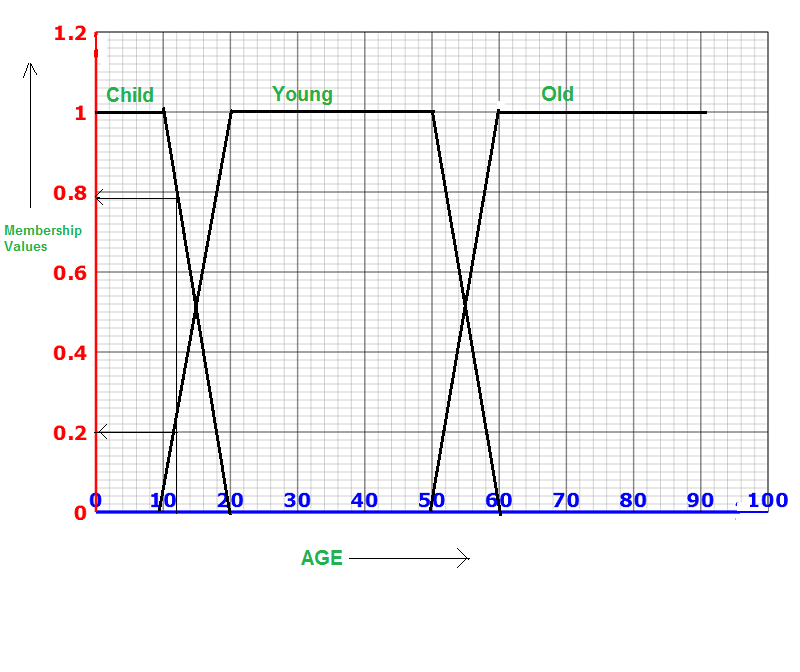# Linguistic variable And Linguistic hedges

• Last Updated : 08 Oct, 2021

Introduction :

To understand the fuzzy logic & fuzzy set theory, it is important to be familiar with the linguistic variable linguistic hedges. Let’s look at both of them one by one to understand the fuzzy set theory better.

Attention reader! Don’t stop learning now. Get hold of all the important Machine Learning Concepts with the Machine Learning Foundation Course at a student-friendly price and become industry ready.

Linguistic Variables :
Variables in mathematics normally take numeric values, although non-numeric linguistic variables are frequently employed in fuzzy logic to make the expression of rules and facts easier. For instance, the term ‘Age’ can be used to indicate a linguistic variable with a value such as a child, young, old, and so on.

Linguistic variables are variables with a value made up of linguistic concepts (also known as linguistic words) rather than numbers, such as child, young, and so on.
Let’s define AGE as a language variable:

`AGE = {Child, Young, Old}`

Each AGE linguistic phrase has a membership function for a specific age range. The same age value is mapped to multiple membership values in the range of 0 to 1 by each function. These membership values can then be used to identify whether a person is a child, a young person, or an elderly person. The following is the membership function with regard to each fuzzy term:Representation of Linguistic Terms of Age

For AGE = 11, we will get a membership value of 0.75 (roughly) in the Child set, 0.2 (approx) in the Young set, and 0 in the Old set, as shown in the diagram. So, if a person’s age is 11, it’s safe to assume that he or she is a child, perhaps a little young but certainly not old.

The formal definition for the linguistic variable is written as :

```x,T(x), U, G, M), where :
x - Variable name (in this case, AGE)
T(x)- Set of linguistic terms such as {Child, Young, Old}
U - Universe
G - Syntactical rules which generate the modifies value
linguistic term of x
M - Semantic rules associated with each linguistic term of x with its meaning.```

Let us consider an example such as (AGE, {Child, Young, Middle-aged, Old} and U – Set of ages of all the people, G, M).
The Semantic rules M give meaning to each linguistic term of AGE. Example: M for the linguistic term Old of the  linguistic variable AGE is defined as :

`M = {(x,μOld(x)| x∈[0,100]} `

and

```μOld(x)    = { 0 ; ≤ 50
= { (x - 50) / 15 ; 50 < x ≤ 65
= { 1 ; x > 65```

The syntactical rules: G that generate the modified value of the linguistic terms of x is given as :

```G = (Vn, VT, Pr, S)where :
Vn - Non Terminal Symbols
VT - Terminal Symbols
Pr - Production Rules
S - Start Symbol```

Example :
Let S be start symbol , Vn = {A, B, C, D, E, S}
VT = {and, or, very, more or less, not, almost, young……}
Pr = Production Rules : S -> A, S -> S or A, A -> A and B etc.

Linguistic hedges :
Linguistic hedges can be used to modify linguistic variables, which is an important feature. Linguistic hedges are primarily employed to aid in the more precise communication of the degree of correctness and truth in a particular statement.
For example: If a statement John is Young is associated with the value 0.6 then very young is automatically deduced as having the value 0.6 * 0.6 = 0.36. On the other hand, not very young get the value ( 1 – 0.36 ), i.e., 0.64. In this example, the operator very(X) is defined as X*X; however, in general, these operators may be uniformly, but flexible, defined to fit the application; this results in great power for the expression of both rules and fuzzy facts.  Linguistic modifiers such as very, more or less, fairly, and extremely rare examples of hedges. They can modify fuzzy predicates and fuzzy truth values.

In general, for a given fuzzy proposition P, such that

`P : x is F`

And a linguistic hedge H, we can construct a modified proposition as

`HP : x is HF`

Here, HF denotes the fuzzy predicate obtained by applying hedge H to predicate F. The predicate can be further modified by applying the hedge to a fuzzy truth value in the given proposition. Any hedge H is interpreted as unary operator ‘h’ on the interval [0,1]. For example hedge very is often interpreted as the unary operator :
h(a) = a2 ; for a ∈ [0,1]
Similarly, hedge fairly interpreted as the unary operator :
h(a) = √a ; or a ∈ [0,1]

A strong modifier strengthens a fuzzy predicate to which it is applied and consequently reduces the truth value of the associated proposition. A weak modifier weakens the predicate and consequently increases the truth value of the associated proposition.
Example: If Young (25) = 0.8, Then : very Young(25) = 0.8 ×0.8 = 0.64 and fairly young(25) = √0.8 = 0.89

The modifier h should satisfy the following conditions :

```- h is a continuous function
- h(0) = 0; h(1) = 1
- If h is strong, then 1/h is weak and vice versa
- If h and g are two modifiers then compositions of these
modifiers are also modifier such as very little.
If both modifiers are strong, then the composition is also strong and vice versa.```
My Personal Notes arrow_drop_up